<< Previous    1...   13  14  15    17    Next >>

The Three Absent Polygons Exhibit the 10×24 Pattern Characteristic of Holistic Systems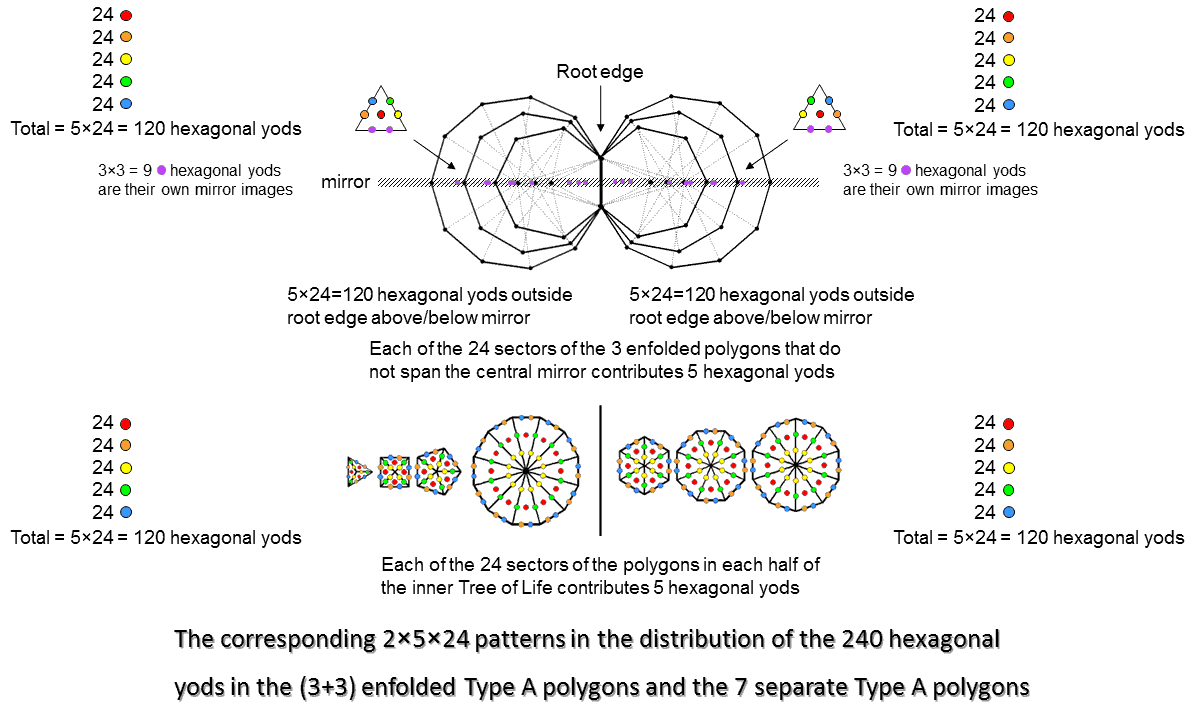The 3 regular polygons absent from the sequence of 7 regular polygons that make up the inner form of the Tree of Life are the heptagon, nonagon & undecagon. When separate, they have 27 sectors with 30 corners & 54 sides. Enfolded, they have 27 sectors with 26 corners (24 outside the root edge), 25 external sides (24 outside the root edge) & 27 internal sides. As tetractyses, the sectors contain (5×27=135) hexagonal yods when separate and (135−2−2=131) hexagonal yods when enfolded (129 outside the root edge). The 3 tetractys sectors sharing the root edge have a hexagonal yod (coloured violet in the diagram) at their centres that lies on the axis passing through the centres of the (3+3) enfolded polygons. Two violet hexagonal yods in each polygon line the internal side that lies on the axis diametrically opposite this hexagonal yod. If we imagine the axis as a mirror, then every yod in the upper half of a polygon has a counterpart in the lower half that is its mirror reflection. But yods lying on the axis are their own mirror images. So each polygon has 3 hexagonal yods that coincide with their mirror images. The number of hexagonal yods outside the root edge in each set of 3 enfolded polygons = 129 − 3×3 = 120. The (3+3) polygons have (120+120=240) hexagonal yods that have distinct mirror images. In each set of 3 polygons, there are 24 external sides outside the root edge, each lined by a pair of hexagonal yods, and 24 internal sides above and below the mirror, each lined by a pair of hexagonal yods. There are 24 tetractys sectors above and below the mirror, each with a hexagonal yod at its centre. The (2+2+1=5) hexagonal yods per tetractys sector are repeated 24 times over all the sectors. The (5+5=10) hexagonal yods per pair of sectors in the (3+3) enfolded polygons are repeated 24 times. These 5×24 & 10×24 patterns are characteristic of sacred geometries. For example, see here, here & Article 53. The 7 regular polygons of the inner Tree of Life have two "halves": the triangle, square, pentagon & dodecagon with 24 sectors and the hexagon, octagon & decagon with 24 sectors. Constructed from tetractyses, the former half has 120 hexagonal yods (5 hexagonal yods per sector repeated 24 times) and the latter half has 120 hexagonal yods (5 hexagonal yods repeated 24 times). The two halves of the 7 types of polygons making up the inner Tree of Life have their counterpart in the two sets of the three absent polygons because both geometries display the 24:24 division in sectors that is characteristic of holistic systems. The (3+3) enfolded polygons actually have (27+27=54) sectors. But only (24+24=48) sectors have distinct mirror images, the 3 sectors in each set that straddle the mirror being mirror images of themselves.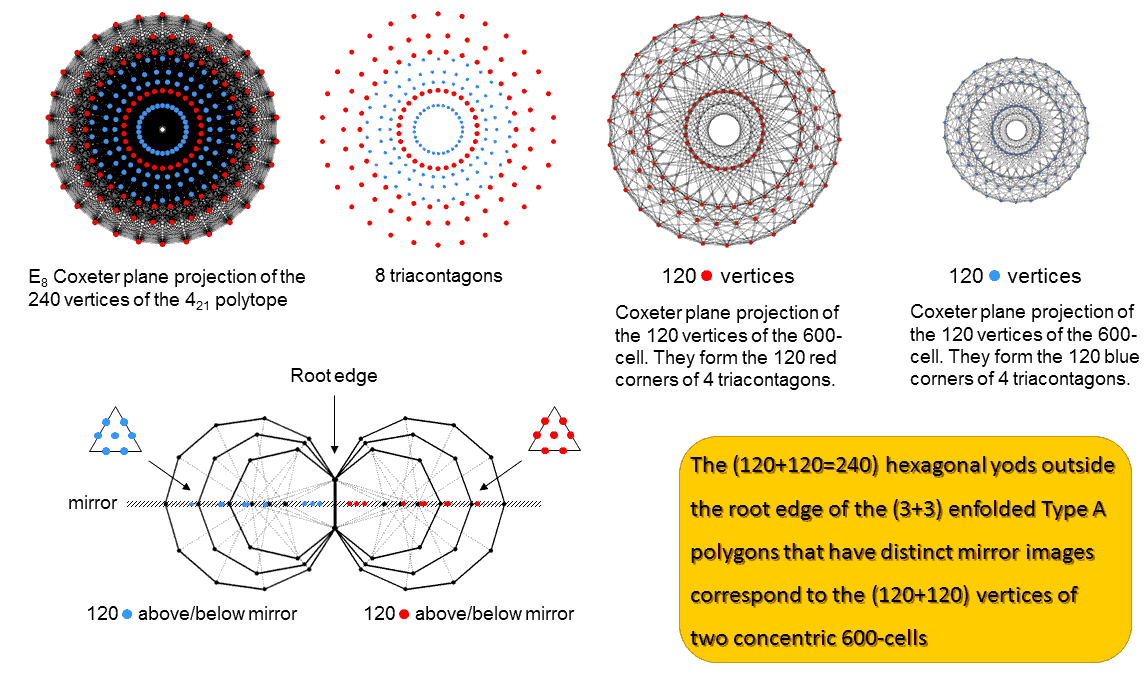Article 62 analyses these 5×24 & 10×24 patterns in the polychoron composition of the 421 polytope. Their counterparts in sacred geometries are discussed in Article 53 and here. The E8 Coxeter plane projection of its 240 vertices consists of 8 concentric triacontagons with 30 corners. Four concentric triacontagons with 120 corners (denoted by red dots) are the H4 Coxeter plane projection of the 120 vertices of a 600-cell. Four smaller concentric triacontagons with 120 blue corners are the H4 Coxeter plane projection of the 120 vertices of a smaller 600-cell. This is because the Coxeter plane projection in 4 dimensions of the 240 vertices of the 421 polytope is a compound of two 600-cells. Amazingly, the (3+3) enfolded polygons display the same 120:120 division in their 240 hexagonal yods above and below their axis. Because of its inversion symmetry, every vertex in the 4-dimensional 600-cell with Cartesian coordinates (x1, x2, x3, x4) has its "opposite," or mirror image, with Cartesian coordinates (−x1, −x2, −x3, −x4). This is the reason for the requirement that every hexagonal yod should have a distinct mirror image; those that do not cannot denote vertices. One set of 3 polygons is the polygonal analogue of the larger 600--cell with 120 red dots representing its vertices and the other set is the counterpart of the smaller 600-cell with 120 blue dots representing its vertices. Their 120 hexagonal yods denote the vertices of each 600-cell. Just as the 60 hexagonal yods outside the root edge in the upper half of a set of 3 enfolded polygons have their mirror image counterparts in the 60 hexagonal yods in the lower half, so the 60 vertices in one half of the 600-cell have their inversions in the 60 vertices of its opposite half. The implication of their conformity to one of the characteristic patterns displayed by sacred geometries, namely, 240 = 120 + 120, is that the two sets of polygons absent from the inner Tree of Life contain, so to speak, the 2-dimensional seeds that grow into the 8-dimensional, 421 polytope whose 240 vertices represent the 240 roots of E8. Here in just 3 types of regular polygons is the beginning of the complete, mathematical symmetry of the forces between E8×E8 heterotic superstrings. The complex geometry of an 8-dimensional object has been reduced to that of three particular polygons because they are the precursor of the 2-polytopic form of the inner Tree of Life, of which the 421 polytope is the 8-polytopic version.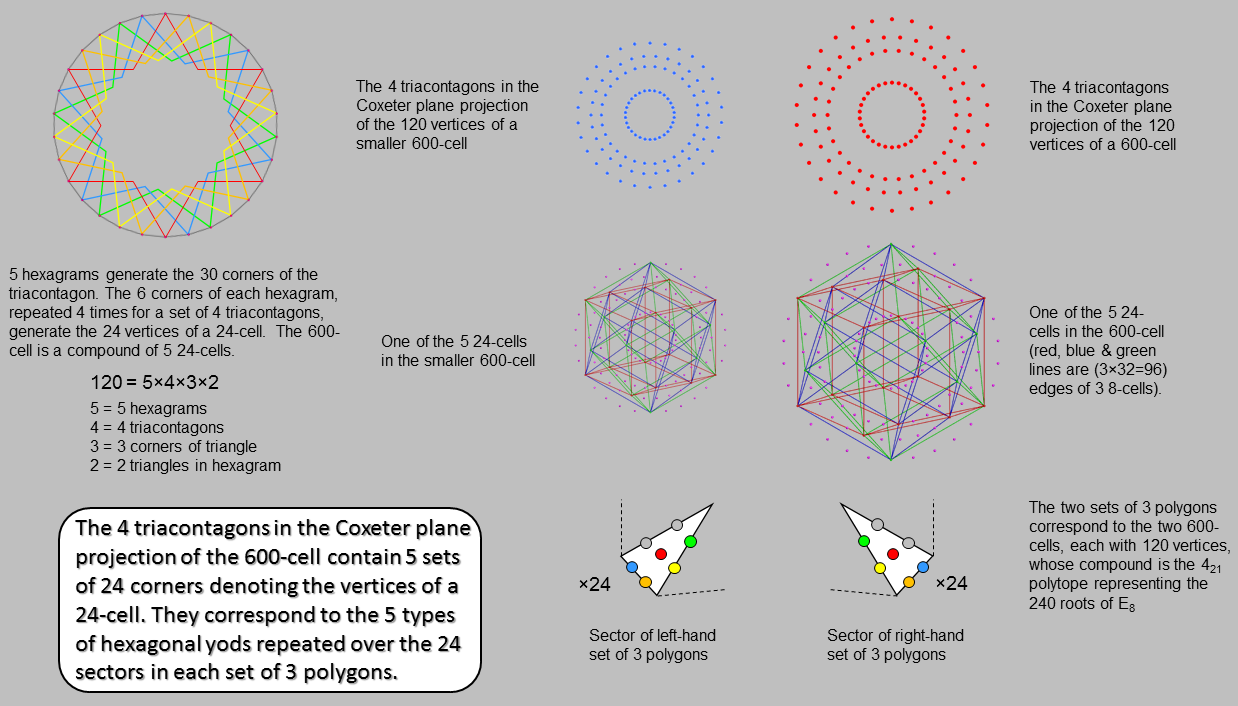As 30 = 5×6, a triacontagon with 30 corners is generated by 5 hexagrams (coloured red, orange, yellow, green & blue), a hexagram being composed of 2 overlapping, equilateral triangles, each with 3 corners. The 4 triacontagons in the H4 Coxeter plane projection of a 600-cell with 120 vertices have 120 corners, where 120 = 5! = 5×4×3×2×1. We see that this factorisation has a simple, geometrical origin. The factor of 5 arises because a 600-cell is a compound of 5 24-cells, each with 24 vertices that correspond to the corners of a single hexagram in each triacontagon. Each 600-cell occupies a 4-dimensional subspace of the 8-dimensional space occupied by the 421 polytope. Its 4-dimensional Coxeter plane projection is a compound of two 600-cells, one smaller than the other. This means that it can be regarded as a compound of (5+5=10) 24-cells. The pair of sets of 3 enfolded polygons with(120+120=240) hexagonal yods is the counterpart of the pair of 600-cells with (120+120=240) vertices. There are 5 hexagonal yods per tetractys sector of a polygon. These 5 types of hexagonal yods are repeated 24 times over the 24 sectors of the heptagon, nonagon & undecagon that have distinct images reflected across the plane of the mirror in their axis. Similarly, the 5 types of hexagonal yods in the sectors of the second set of 3 polygons are repeated 24 times. Each type of hexagonal yod symbolises a vertex of one of the 5 24-cells. Its 24 repetitions correspond to the 24 vertices of a particular 24-cell. The 5:5 division of hexagonal yods and 24-cells corresponds in the outer Tree of Life to its 5 uppermost Sephiroth and its 5 lowest Sephiroth. Article 53 discusses the 10-fold (or, rather, double 5-fold) division in various sacred geometries. Article 62 discusses their relevance to the 10 disjoint 24-cells in the 421 polytope. The 120:120 division in the hexagonal yod populations of the (3+3) polygons is a characteristic pattern of all sacred geometries. It applies to the 24-cell itself, which has 24 vertices, 96 edges, 96 triangular faces & 24 octahedral cells. Its 240 0-, 1-, 2- & 3-polytopes comprise 120 vertices & edges and 120 faces & cells (see here). Its Petrie polygon is the dodecagon, the last in the sequence of the first 10 regular polygons. This confirms the holistic character of the 24-cell, which should come as no surprise, given that the 5:5 pattern of 24-cells in the Coxeter plane projection of the 421 polytope as a compound of two 600-cells is just the polytopic manifestation of the 5:5 division of the 10 Sephiroth of the Tree of Life.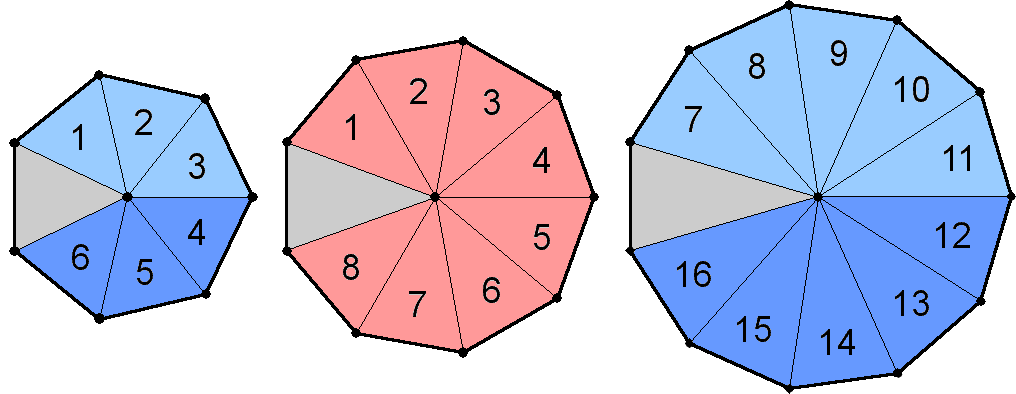24 = 8 + 16 = 8 + (3+5=8) + (3+5=8) 24 → 24 transverse dimensions of bosonic string. Nonagon: 8 → 8 transverse dimensions of superstring. Heptagon+undecagon: 16 → 16 bosonic string dimensions. Signature of transverse string dimensions in the 3 absent polygons The grey sectors of the 3 polygons shown opposite are their own mirror images when reflected in the mirror lying in the axis joining their centres. The 24 sectors of each set of 3 polygons that have distinct mirror images comprise 6 in the heptagon (light blue & dark blue), 8 in the nonagon (red) and 10 in the undecagon (light blue & dark blue), i.e., 24 = 6 + 8 + 10. The counterpart of this in the 7 polygons with 48 sectors that make up the inner Tree of Life is that one of its "halves"* consists of the hexagon with 6 sectors, the octagon with 8 sectors and the decagon with 10 sectors. The 8:16 division appears also in the other half because the triangle & pentagon have 8 sectors, whilst the square & dodecagon have 16 sectors. The theory of spinless strings (so-called "bosonic strings") requires space-time to be 26-dimensional, so that they have 24 transverse dimensions, Superstring theory requires superstrings to have 8 transverse dimensions. As hybrids of superstrings and bosonic strings, heterotic superstrings have left-moving and right-moving excitations that are completely decoupled. The left-moving (anticlockwise) excitations are bosonic strings moving in 26 dimensions, whilst the right-moving (clockwise) excitations are 10-dimensional superstrings. Because of the isomorphism between N overlapping Trees of Life and the N tetractys sectors of an N-gon (see here), the 24 sectors of either the 3 polygons absent from the inner Tree of Life or each half of the set of 7 polygons that comprise it can be interpreted in terms of 24 overlapping Trees of Life. If these sectors can also denote dimensions of space transverse to the direction of motion of a string, then these Trees of Life can be understood as signifying such dimensions. It would indicate that the nonagon with 8 distinct, mirror-image sectors should be associated with the 8 transverse dimensions of superstrings and with the 8 dimensions of the root vector space of E8, whilst the heptagon & undecagon with 16 distinct sectors should be associated with the 16 higher, bosonic string dimensions. Notice that the 8 light blue sectors in the upper halves of these two polygons are mirrored by 8 dark blue sectors in their lower halves. This means that the 24 sectors of the 3 polygons naturally divide into 3 sets of 8. The significance of this is revealed below. As the 3 enfolded polygons have 27 sectors with 26 corners (24 outside the root edge), their shapes symbolise the 26 dimensions of bosonic strings. The two endpoints of the root edge denote time and the longitudinal dimension, the 8 corners of the 9 sectors of the nonagon outside it denote the 8 transverse, superstring dimensions and the (6+10=16) corners of the 18 sectors of the heptagon & undecagon outside the root edge denote the 16 transverse, bosonic string dimensions. * The two halves of the inner Tree of Life are discussed in Section 2 of Article 64.

There is another way in which the 8:16 division displayed by the 3 polygons absent from the inner Tree of Life manifests in heterotic superstring theory. Taking the edge length of the 24-cell as 1, eight of its vertices have coordinates that are all the permutations of (±1, 0, 0, 0); they are vertices of the 16-cell: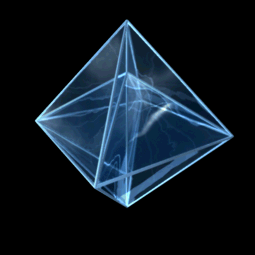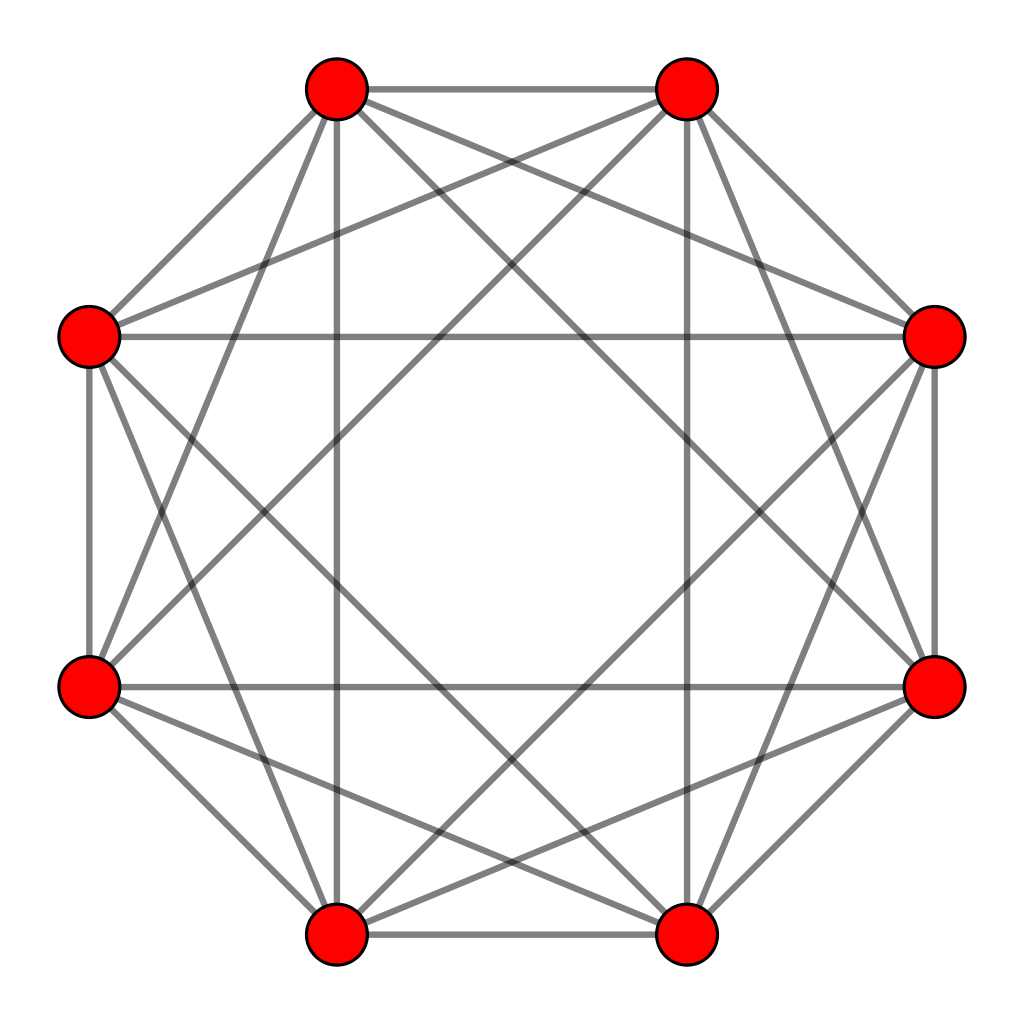Isometric Orthogonal Projection of: 8-Cell (Tesseract) + 16-Cell = 24-Cell. Credit: Title: Cell24Construction.ogv Author: Jgmoxness The rotating 16-cell. Petrie polygon of the 16-cell.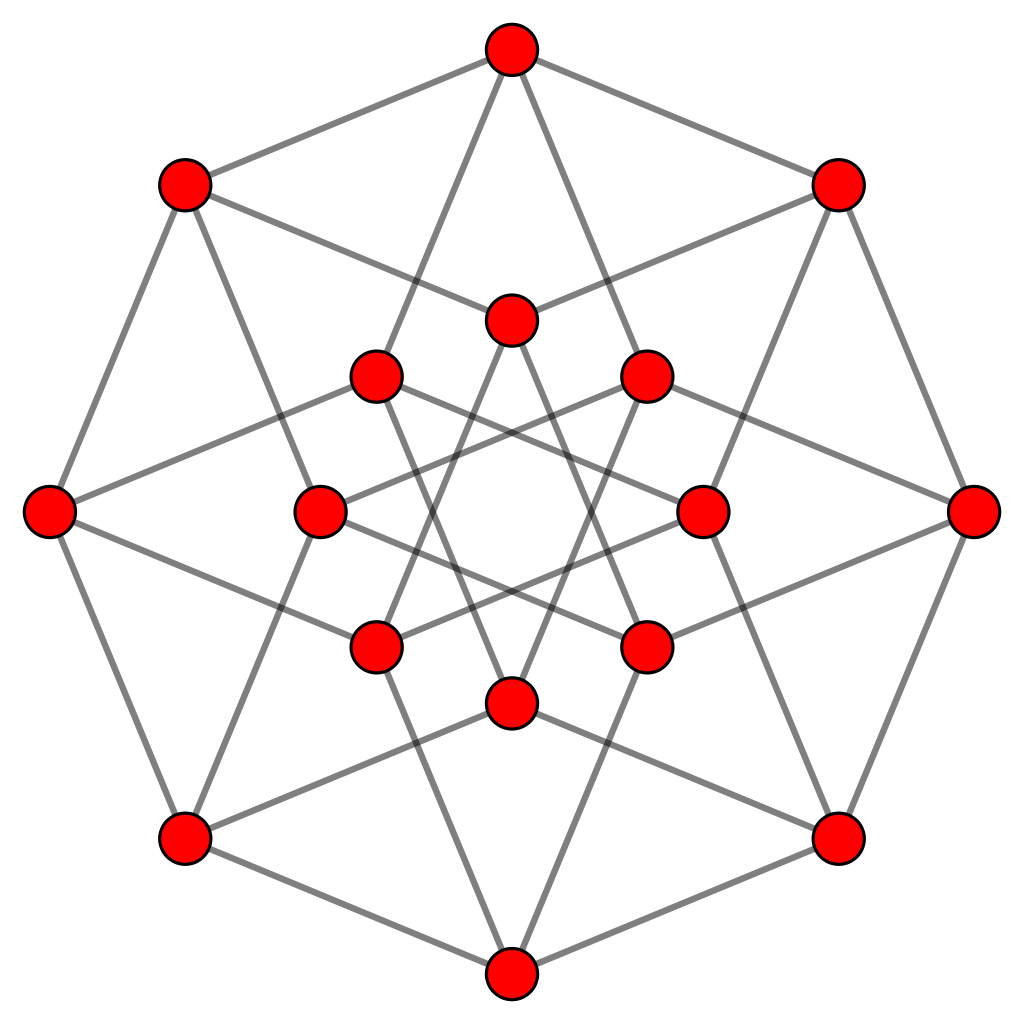The rotating 8-cell. Graph of the 8-cell.

Sixteen vertices of the 24-cell are of the form: (±½, ±½, ±½, ±½); they are the vertices of an 8-cell. They can be divided into two groups of eight: those with an even number of (−) signs and those with an odd number of (−) signs. Each group of vertices defines a 16-cell. Therefore, the 24 vertices of a 24-cell can be grouped into three sets of eight, each set defining a regular 16-cell. This is where the analogy with the sectors of the three polygons appears to break down, for 16 = 6 + 10 in their case, instead of 16 = 8 + 8 for the 8-cell. However, the upper halves of the heptagon & undecagon have (3+5=8) sectors, as do their lower halves. Therefore, the analogy holds up because each of the two halves of these two polygons in combination has 8 sectors, those in one half being the mirror images of those in the other half. Just as the 24 vertices of the 24-cell do, the 24 sectors with mirror-image counterparts divide up into three sets of 8:

1. the 8 sectors of the nonagon that are mirror images of one another → 8 vertices of the 16-cell → 8 transverse dimensions of the superstring;
2. the 8 upper sectors of the heptagon & undecagon → 8 vertices of a 16-cell → 8 higher, transverse dimensions of bosonic string excitations of the heterotic superstring;
3. the 8 lower sectors of the heptagon & undecagon → 8 vertices of another 16-cell → 8 higher, transverse dimensions of bosonic string excitations of the heterotic superstring.

The 24-cell is a compound of three 16-cells: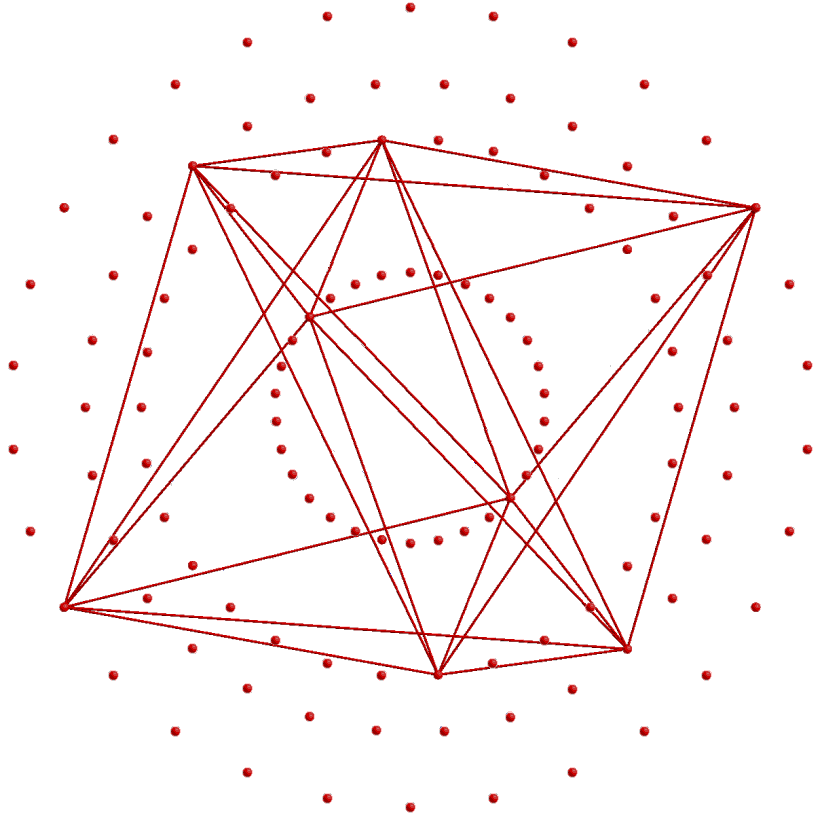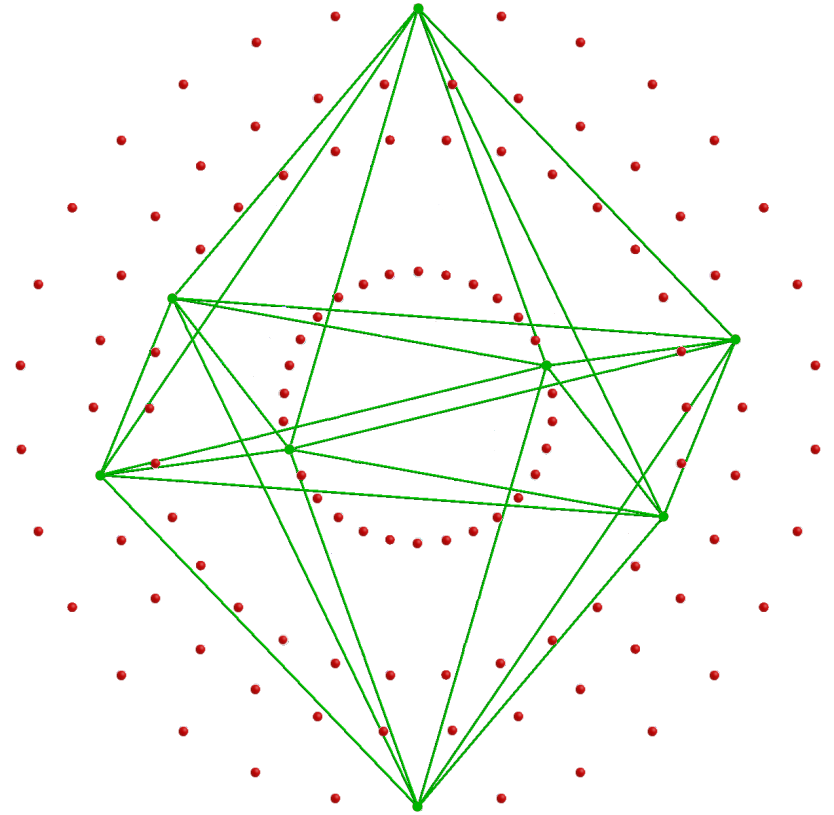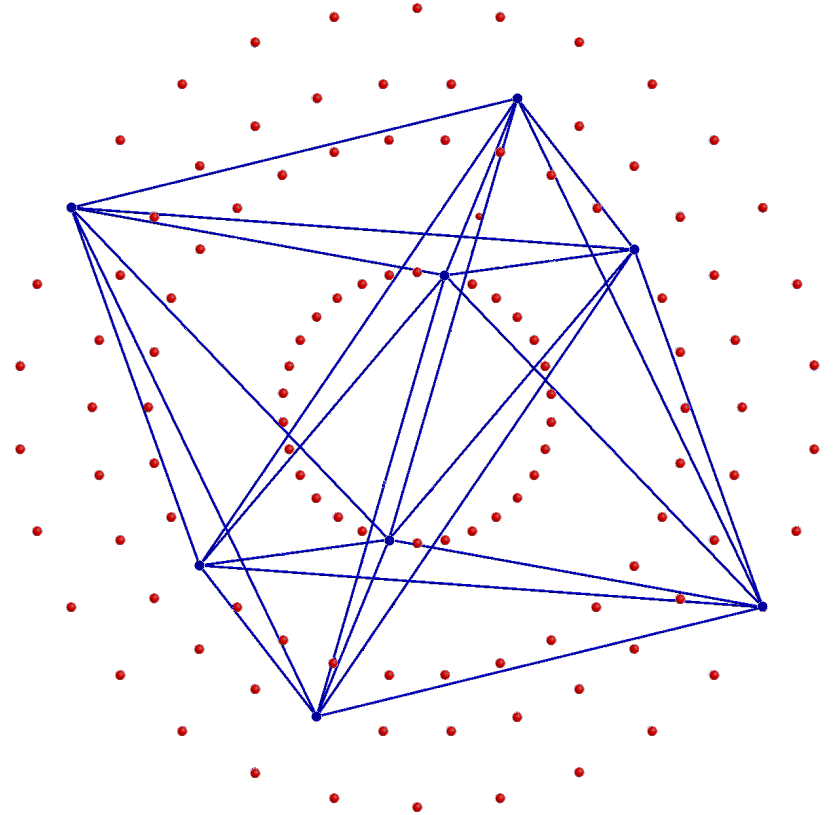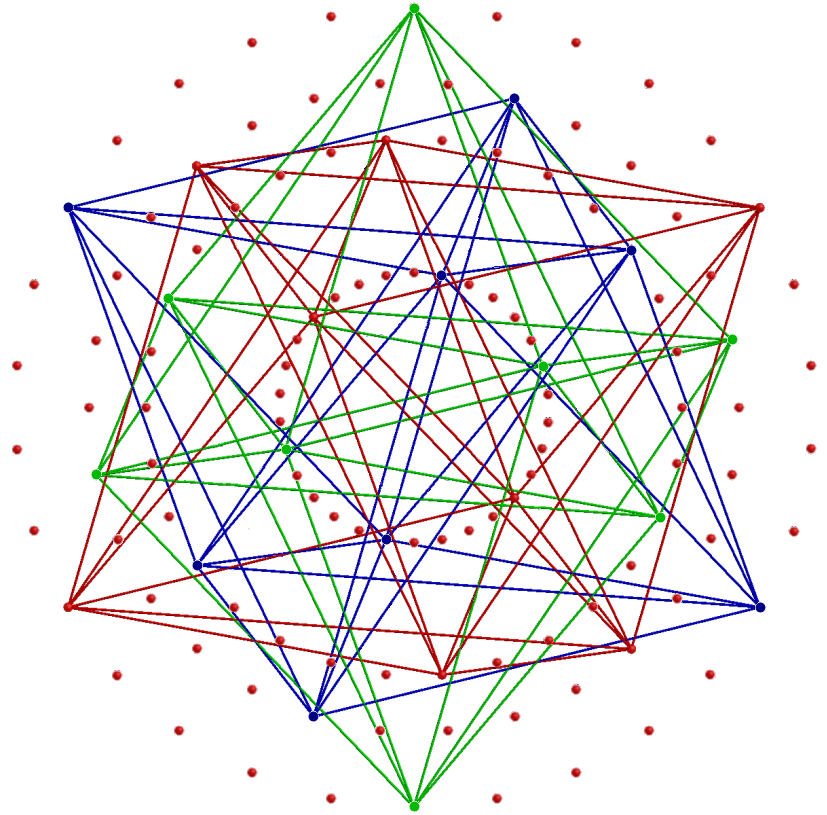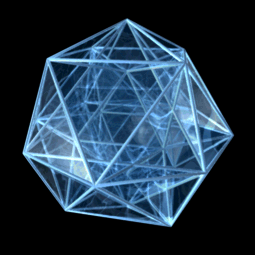A 16-cell in one of the 10 24-cells. A second 16-cell in this 24-cell. A third 16-cell in this 24-cell. The 24-cell as a compound of 3 16-cells. The rotating 24-cell.

The vital property of the three polygons that creates the analogy with the three 16-cells in a 24-cell is that two of the polygons have exactly twice as many sectors (namely, 16) with distinct mirror images as the third (namely, 8). It is, of course, each type of hexagonal yod in the 24 sectors that signifies a vertex of one of the five 24-cells. Only one of the three polygons (the nonagon) is uniquely associated with a 16-cell. But the upper and lower halves of the two other polygons in combination, each with 8 distinct sectors, define second and third 16-cells in the 24-cell associated with a particular type of hexagonal yod. See here for a discussion of the properties of the 8-cell, 16-cell & 24-cell.

The holistic character of the 24-cell as a compound of 3 16-cells
The 16-cell has 8 vertices (a pair along each of the 4 coordinate axes at ±1), 24 edges, 32 triangular faces & 16 tetrahedral cells. It comprises 64 points, lines & triangles and 80 points, lines, triangles & tetrahedra. 64 is the number value of Nogah, the Mundane Chakra of Netzach, and 80 is the number value of Yesod, the penultimate Sephirah of the Tree of Life. Three disjoint 16-cells are composed of (3×64=192) points, lines & triangles and (3×80=240) 0-, 1-, 2- & 3-polytopes. Their 192 geometrical elements comprise (3×8=24) points (12 with positive coordinates, 12 with negative coordinates) and [3×(24+32) = 3×56 = 168] lines & triangles. As shown above, three 16-cells with 24 vertices are associated with each set of three polygons. The division:

192 = 24 + 168

that applies to either 24-cell and the division:

384 = 192 + 192 = (24+24) + (168+168) = 48 + 336

that applies to the 48 vertices and 336 edges & faces of both 24-cells is the basic characteristic of all holistic systems (see The holistic pattern). It is found in sacred geometries and in the 64 hexagrams of the I Ching system of divination: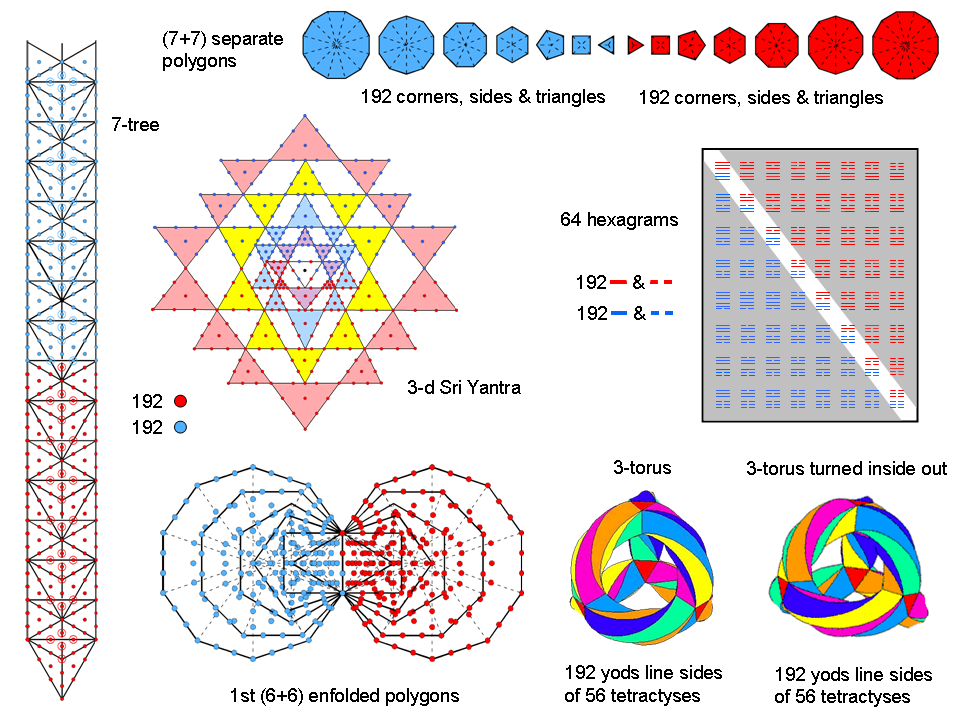The division: 384 = 192 + 192 in various sacred geometries.

Just to take two examples:

• the (24+24=48) lines & broken lines in the 8 diagonal hexagrams of the 8×8 array of 64 hexagrams correspond to the (24+24=48) vertices of the pair of 24-cells associated with both sets of 3 enfolded polygons, namely, the 24-cell and its dual, which is the same polytope: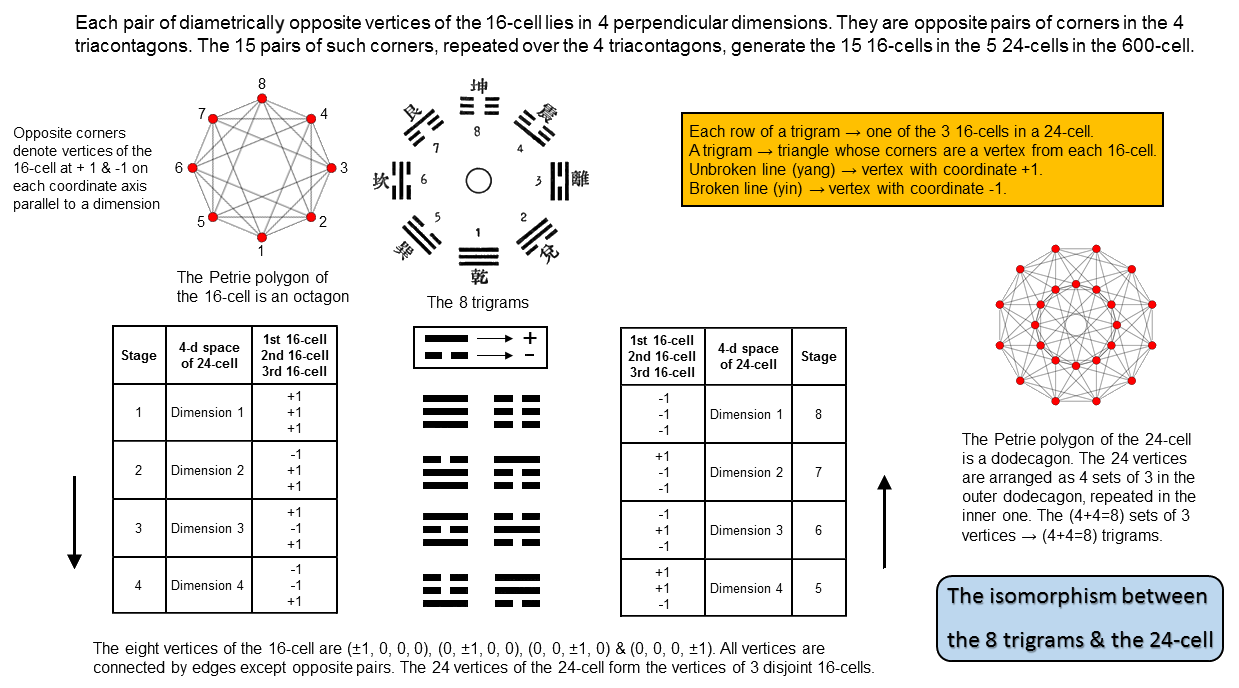The (168+168=336) lines & broken lines in the (28+28=56) off-diagonal hexagrams correspond to the (168+168=336) edges & faces of the two sets of 3 16-cells in the 24-cell and its dual, each cell having 56 edges & faces. The three rows of lines or broken lines in a trigram correspond to the presence of 3 16-cells in the 24-cell. The 56 trigrams above the diagonal correspond to the 56 edges & faces in each 16-cell of the 24-cell and the 56 trigrams below the diagonal correspond to the 56 edges & faces in each 16-cell of the 24-cell associated with the mirror-image set of 3 enfolded polygons. Each of the 384 lines and broken lines in the 64 hexagrams corresponds to one of the 384 vertices, edges & faces in the two sets of 3 16-cells. Each diagonal half with 192 lines & broken lines corresponds to a 24-cell and its dual, each having 3 16-cells with 192 vertices, edges & faces;

• Each of the two sets of the 7 separate Type A polygons of the inner Tree of Life has 192 corners, sides & triangles surrounding their centres. They comprise the 24 corners, sides & triangles surrounding the centre of the hexagon and the 168 corners, sides & triangles surrounding the centres of the six other polygons.

Discussion of the other examples shown in the diagram can be found in the section Correspondences and in #36 of Wonders of Correspondences.

The E8 Coxeter projection of the 421 polytope is that of a compound of two 600-cells. Each 600-cell is a compound of 5 24-cells and each 24-cell consists of 3 16-cells, so that the 600-cell contains 15 16-cells. Each 16-cell has 8 vertices, 24 edges & 32 faces, so that the 15 16-cells contain 120 vertices, 360 edges & 480 faces, i.e., 840 edges & faces. The two 600-cells have 30 16-cells with 240 vertices and 1680 edges & faces, i.e., 1920 vertices, edges & faces. 720 edges join the 120 vertices of each 600-cell, so that it has 840 vertices & edges, which means, remarkably, that two separate 600-cells contain 1680 vertices & edges. The superstring structural parameters 840 & 1680 determined by C.W. Leadbeater for the UPA (see here) quantify the geometrical composition of both the two 600-cells and their 16-cells that make up the 421 polytope representing the unified gauge symmetry group of heterotic superstring forces. Equally remarkable is the fact that these numbers also measure their yod compositions. If we imagine the 30 16-cells constructed from tetractyses, the number of yods lining their (30×24=720) edges = 240 + 720×2 = 1680 (840 in each set of 15). Discounting as implausible the possibility that appearances in three closely related contexts of two paranormally-derived numbers could be due to chance forces us to accept that these mathematical properties are convincing evidence that the UPA is a heterotic superstring that is shaped by the very forces that it exerts, some of which have yet to be discovered because they operate only within quarks. Furthermore, because holistic systems (apart from possible factors of 10) exhibit the division:

384 = 192 + 192,

where

192 = 24 + 168,

(see The holistic pattern), the fact that the two 600-cells have 240 vertices & 1680 edges & faces, i.e., 1920 vertices, edges & faces, implies that the complete, holistic system must comprise two 421 polytopes, so that the symmetry group for superstrings must be E8×E8. The master holistic pattern established in this website to be embodied by sacred geometries requires this direct product structure. The two mirror-image halves of the inner form of the Tree of Life, each encoding E8, are a simple illustration of this (e.g., as one of the many examples discussed elsewhere, see here). One 16-cell has 64 vertices, edges & faces. The three 16-cells in a 24-cell have 24 vertices and 168 edges & faces, i.e., 192 vertices, edges & faces. Because they embody the holistic parameter 192, which is half of 384, they represent half of a holistic system. The 7 regular polygons making up half the complete, inner form of the Tree of Life have 192 geometrical elements surrounding their centres (24 in the hexagon, 168 in the 6 other polygons). The three 16-cells, whose 24 vertices correspond to the 24 corners of sectors outside the root edge of the three enfolded polygons that are absent from the inner Tree of Life, must be complemented by the three 16-cells in the mirror-image set of three enfolded polygons. They make up the dual of the 24-cell, which is just a different configuration of another 24-cell.

 << Previous    1...   13  14  15    17    Next >>

Home